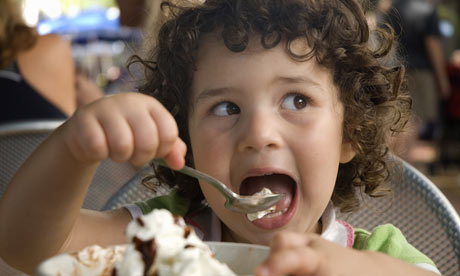# Roopesh - The ice cream eater

Algebra Level 3Roopesh went to his friends house. There he ate a lot of Ice-cream. First he started with Vanilla then Chocolate then Vanilla then Butterscotch then Vanilla. He is a mathematician and made a monic polynomial of degree 5 which gave him values of the first letter of the Ice Cream.

This is the way his polynomial proceeded :-
$p(1) = 22$
$p(2) = 3$
$p(3) = 22$
$p(4) = 2$
$p(5) = 22$

If the value of $p(6) = \overline{abc}$. The first letter of the ice cream he eats would be $a+b+c$. Which ice cream will be eat next?

Assumption: $\overline{abc}$ represents a 3 digit number.

×

Problem Loading...

Note Loading...

Set Loading...